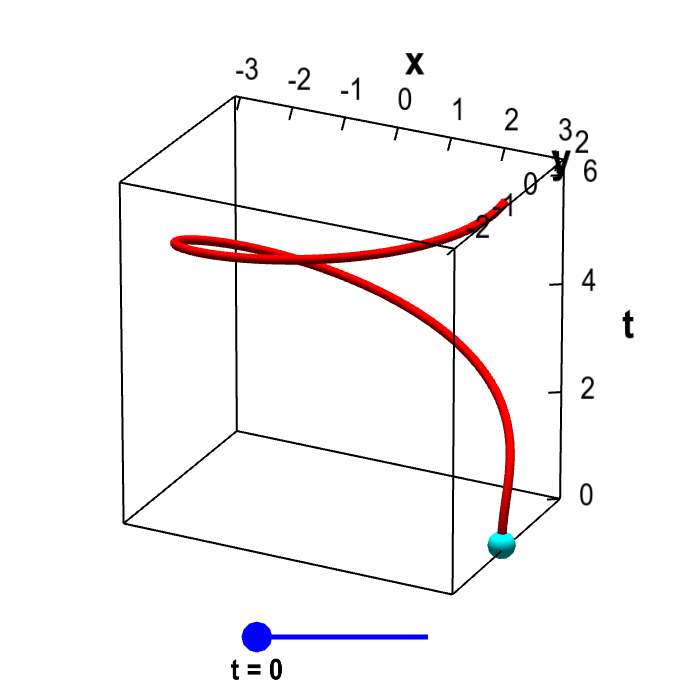# Math Insight

### Applet: Parametrized elliptical helixThe vector-valued function $\sadllp(t)=(3\cos \frac{t^2}{2\pi})\vc{i} + (2 \sin \frac{t^2}{2\pi}) \vc{j}+ t \vc{k}$ parametrizes an elliptical helix, shown in red. This helix is the image of the interval $[0,2\pi]$ (represented by the blue slider) under the mapping of $\sadllp$. For each value of $t$, the cyan point represents the vector $\sadllp(t)$. As you change $t$ by moving the blue point along the interval $[0,2\pi]$, the cyan point traces out the helix.